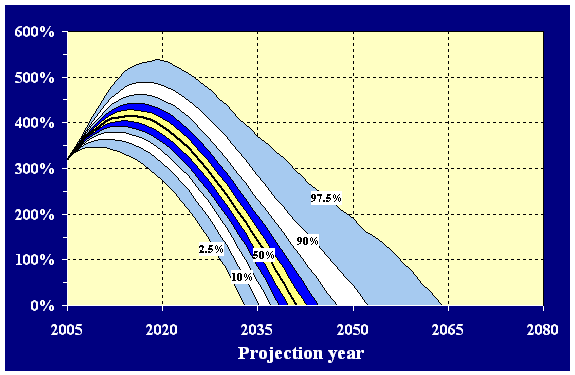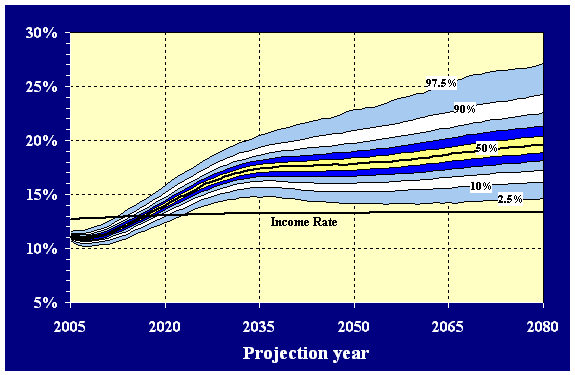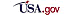## 2005 OASDI Trustees ReportVI. APPENDICES

### E. STOCHASTIC PROJECTIONS

Significant uncertainty surrounds the estimates under the intermediate assumptions, especially for a period as long as 75 years. This appendix presents a way to illustrate the uncertainty of these estimates. It is intended to supplement the traditional methods of examining such uncertainty and to illustrate the potential value of new techniques.

### 1. Background

The Trustees Report has traditionally shown additional estimates using a low cost and a high cost set of specified assumptions to reflect the presence of uncertainty. These additional estimates provide a range of possible outcomes for the projections. However, they provide no indication of the probability that actual future experience will be inside or outside the range of these estimates. This appendix presents the results of a model, based on stochastic modeling techniques, that estimates a probability distribution of future outcomes of the financial status of the combined OASI and DI Trust Funds. It should be noted that this model is in its early stages of development. Future improvements and refinements to the model are expected. In particular, future revisions are expected to reflect a fuller range of uncertainty about the future, as is discussed below.

### 2. Methodology

Other sections of this report provide estimates of the financial status of the combined OASI and DI Trust Funds using a "deterministic" model. For the deterministic model, certain assumptions are made regarding levels of fertility, changes in mortality, immigration levels, emigration levels, net other immigration levels, the Consumer Price Index, average real wages, unemployment rates, trust fund real yield rates, and disability incidence and recovery rates. Each of these variables will reach an assumed ultimate value at a specific point during the long-range period and will maintain that value throughout the remainder of the period. As mentioned above, three deterministic scenarios are developed assuming separate, specified values for each of these variables.

In contrast, the results of 5,000 independent stochastic simulations are presented in this appendix. Each of the 5,000 simulations is determined by allowing the above variables to vary throughout the long-range period. The fluctuation in the variable is projected by using standard time-series modeling, a method designed to help make inferences based on historical data. Generally, each variable is modeled by an equation that captures a relationship between current and prior years' values of the variable and introduces year-by-year random variation, as reflected in the historical period. For some variables, the equations additionally reflect relationships with other variables. Parameters for the equations are estimated using historical data for periods ranging from 20 years to 103 years depending on the nature and quality of data available. More detail on this model, and stochastic modeling in general, is available on the internet.1 Each time-series equation is designed such that, in the absence of random variation, the value of the variable would equal the value assumed under the intermediate set of assumptions.

For each simulation of the model, values of the variables listed above are determined by using Monte Carlo techniques to randomly assign the year-by-year variations. Each simulation produces an estimate of the financial status of the combined OASI and DI Trust Funds. Results shown in this section, based on the 5,000 simulations of the model, reflect the distribution of results.

The results from this model should be interpreted with caution and with a full understanding of the inherent limitations. Results are very sensitive to equation specifications, degrees of interdependence among variables, and the historical periods used for the estimates. For some variables, using the variations exhibited in a relatively recent historical period may not provide a realistic representation of the potential variation for the future. In addition, results would differ if random variations had been applied to additional variables other than those mentioned above (such as labor force participation rates, retirement rates, marriage rates, and divorce rates). Furthermore, additional variability could result from incorporating statistical approaches that would more fully model change in the long-range central tendencies of the variables. The historical period available for most variables is relatively homogeneous and does not reflect many substantial shifts. The time-series modeling reflects what occurred in the historical period. As a result, the variation indicated in this appendix should be viewed as the minimum plausible variation for the future. Substantial shifts, as predicted by many experts and as seen in prior centuries, are not fully reflected in the current model.

### 3. Results

Simulated probability distributions of the annual trust fund ratios for the combined OASI and DI Trust Funds are shown in figure VI.E1. The two extreme lines in this figure illustrate the range within which future annual trust fund ratios are estimated to occur 95 percent of the time (i.e., a 95-percent confidence interval). In other words, actual future trust fund ratios in a given year would be expected to exceed the upper bound only 2.5 percent of the time or to fall below the lower bound 2.5 percent of the time. Other lines in the figure display additional confidence intervals (80-percent, 60-percent, 40-percent, and 20-percent) around future annual trust fund ratios. The median estimate for each year indicates the trust fund ratio which is projected by this model to fall exactly in the middle of possible outcomes for that year. It is important to note that these lines do not represent the results of individual stochastic simulations. Instead, for each given year, they represent the percentile distribution of trust fund ratios based on all stochastic simulations for that year.

The median estimate for each year indicates that the assets of the combined OASI and DI Trust Funds would be exhausted by 2041 with a probability of 50 percent. This exhaustion date is the same as the year of exhaustion projected under the intermediate assumptions. Figure VI.E1 shows that the 95-percent confidence interval for the trust fund ratio in 2030 ranges from 439 to 80 percent of annual cost. In comparison, the 2030 trust fund ratios for the low cost and high cost alternatives are each outside this range, at 493 and 10 percent, respectively. By 2079, the range represented by the low cost and high cost projections increases substantially beyond the boundaries of the 95-percent stochastic confidence interval, as seen from the values for the open group unfunded obligation in table VI.E1. This increased variation of the alternatives relative to the stochastic confidence interval is also seen in the positive trust fund ratio for the low cost scenario for 2079.[D]

The probability distribution of the year-by-year OASDI cost rates (i.e., cost as a percentage of taxable payroll) is shown in figure VI.E2. The range of the cost rates widens as the projections move further into the future, reflecting increasing uncertainty. The income rate under the intermediate assumptions is also included in the figure in order to give some indication of the patterns of cash flow for the OASDI program. Only this income rate is included because of the relatively small variation in income rates throughout the projection period. The lines in the figure display the median set (50th percentile) of estimated annual cost rates and the 95-percent, 80-percent, 60-percent, 40-percent, and 20-percent confidence intervals expected for future annual cost rates. It is important to note that these lines do not represent the results of individual stochastic simulations. Instead, for each given year, they represent the percentile distribution of cost rates based on all stochastic simulations for that year. The projected cost rates for the year 2035 for the low cost and high cost alternatives described earlier are 14.80 percent of payroll and 20.22 percent of payroll, respectively. These are quite close to the limits of the 95-percent confidence interval, as seen in figure VI.E2. By 2079, the cost rates for these alternatives, 13.84 and 26.76 percent of payroll, are still fairly close to the limits of the 95-percent confidence interval (14.54 and 26.94 percent of payroll).[D]

Table VI.E1 displays long-range actuarial estimates for the combined OASDI program resulting from using both the deterministic and stochastic approaches. Actuarial estimates included in the table are for the long-range period, 2005-79. Stochastic estimates are shown for the median (50th percentile) and for the 95-percent and 80-percent confidence intervals. For comparison, deterministic estimates are shown for the intermediate, low cost, and high cost assumptions. Each stochastic estimate displayed in the table does represent the results of one stochastic simulation. However, for a given percentile, the stochastic estimates shown for the different long-range actuarial measures are generally not from the same stochastic simulation.

Median stochastic estimates for the actuarial measures displayed in table VI.E1 are the same or slightly more pessimistic for the combined OASI and DI Trust Funds than those projected under the intermediate assumptions. The median estimate of the long-range actuarial balance is -2.01 percent of taxable payroll, about 0.09 percentage point lower than projected under the intermediate assumptions. The median estimate for the first year cost exceeds tax income is 2017 and for the year assets first become exhausted is 2041. These are the same as those projected under the intermediate assumptions. The median estimate for the annual cost in the 75th year of the projection period is 19.55 as a percent of taxable payroll and 6.55 as a percent of GDP. The comparable estimates using the intermediate assumptions are 19.08 and 6.39, respectively.

The 95-percent confidence interval determined by the stochastic modeling projections can be compared to the range of variation defined by the traditional low cost and high cost alternatives. For three of the measures in table VI.E1 (the actuarial balance, the open group unfunded obligation, and the year assets become exhausted), the 95-percent stochastic projection range is narrower than the range defined by the low cost and high cost alternatives. That is, for these measures, the estimates under the low cost and high cost alternatives fall outside the 95-percent confidence interval determined by the stochastic modeling projections. In contrast, for two other measures in the table (the first year cost exceeds tax income and the annual cost in the 75th year of the projection period expressed as a percent of GDP), the 95-percent stochastic projection range includes the estimates under the low cost and high cost alternatives. For the remaining measure in the table (the annual cost in the 75th year of the projection period expressed as a percent of taxable payroll), the 95-percent stochastic projection range includes the estimate under the high cost alternative, but does not include the low cost estimate.

Table VI.E1.—Long-Range Estimates Relating to the Actuarial Status of the Combined OASDI Program

[Comparison of deterministic and stochastic results]

Traditional
deterministic model

Stochastic model
Interme-
diate
Low
Cost
High
Cost
Median
50th
percentile

80-Percent
confidence interval

95-Percent
confidence interval
10th
percentile
90th
percentile
2.5th
percentile
97.5th
percentile
Actuarial balance
-1.92
0.38
-4.96

-2.01

-3.15
-0.99

-3.83
-0.49
Open group unfunded
obligation (in trillions)
\$4.0
-\$1.1
\$10.9

\$4.2

\$7.0
\$1.9

\$8.8
\$0.8
First year cost exceeds tax income
2017
2022
2013

2017

2014
2020

2013
2022
Year assets become exhausted
2041
2030

2041

2035
2052

2032
2064
Annual cost in 75th year
(percent of taxable payroll)
19.08
13.84
26.76

19.55

16.10
24.15

14.54
26.94
Annual cost in 75th year (percent of GDP)
6.39
5.01
8.26

6.55

5.39
8.09

4.87
9.02
 1The fund is not estimated to be exhausted within the projection period.

1 The internet address is: www.socialsecurity.gov/OACT/stochastic/index.html.Privacy Policy | Website Policies & Other Important Information  | Site Map  | Actuarial Publications 3/23/2005